# Basic functions - math word problems

1. Two sisters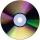Two sisters together have 54 CDs. 6/8 CDs has younger sister and is equal to 3/5 of CDs older sisters. How many CDs has each of the sisters?
2. Acid solutionBy adding 250 grams of a 96% solution of sulfuric acid to its 3% solution its initial concentration was changed to 25%. How many grams of 3% of the acid were used for dilution?
3. WineA bottle of wine costs 21 euros; and wine is 20 times more expensive than a bottle. How much a bottle cost?
4. Unknown number 24I think the number: a - is the same as the square area that has the 12th circumfence. What is this number? b - its half is 7 times bigger than its quarter. Is this the number?
5. Identity123456789 = 100 Use only three plus or minus characters to correct previous identity/equation.
6. Washing mashine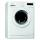Family buy a washing machine for 350e. Cash paid 280e. What percentage of the total price must still pay the washer?
7. TrucksThree lorries droved bricks. One drove n bricks at once, second m less bricks than the first and third 300 bricks more the first lorry. The first lorry went 4 times a day the largest went 3 times a day and the smallest 5 times a day. How many bricks br
8. ClassroomOne-eighth of 9th class was interested in studying at a grammar school, at a business academy one sixth, at secondary vocational schools quarter, to SOU one third and the remaining three students were interested in the school of art direction. How many st
9. Kaufland sales-off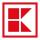As a rule the goods to the approaching end consumption in Kaufland three days before the end of the consumption to 30% off and one day before the end of the consumption to 50% discounts. Sometimes however the seller has been mistaken and the second discoun
10. Enterprise company30% of all employees in the enterprise are women. Men are 360 more. How many employees are in the company?
11. Sum of moneyOn a certain sum of money, invested at the rate of 10% compounded annually, the difference between the interests of the first year and the third year is 315 . Find the sum.
12. Ten fractionsWrite ten fractions between 1/3 and 2/3
13. Unknown number 16My number's tens is three times more then ones My number's ones is twice the number of thousands and my number's hundreds is half the number of of tens. I have two ones. which number am I?
14. DividendsThe three friends divided the win by the invested money. Karlos got three eighths John 320 permille and the rest got Martin. Who got the most and which the least?
15. ChocolateChildren break chocolate first to third and then every part of another half. What kind got each child? Draw a picture. What part would have received if each piece have halved?
16. Sales off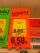shoes cost x euros. First they are discounted by 50%, and then 25% of the new amount. Determine what percentage of the total cost is cheaper.
17. Two acesFrom a 32 card box we randomly pick 1 card and then 2 more cards. What is the probability that last two drawn cards are aces?
18. Table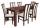The circumference of the rectangle table is 420 cm. Length to width ratio is 5:2 . Calculate table dimensions and dimensions reduced in the ratio 3:5
19. Unknown number 6Determine x if 1/6 of x is equal to 2/5 of the number 24.
20. Trapezoid - central medianThe central median divides the trapezoid into two smaller trapezoids. Determines the ratio of their contents.

Do you have an interesting mathematical word problem that you can't solve it? Enter it, and we can try to solve it.

To this e-mail address, we will reply solution; solved examples are also published here. Please enter the e-mail correctly and check whether you don't have a full mailbox.

Please do not submit problems from current active competitions such as Mathematical Olympiad, correspondence seminars etc...